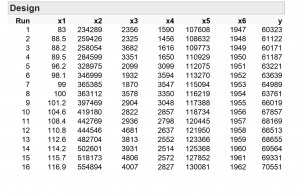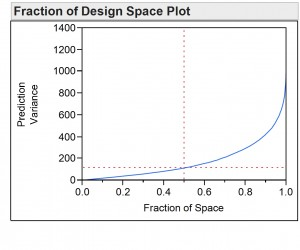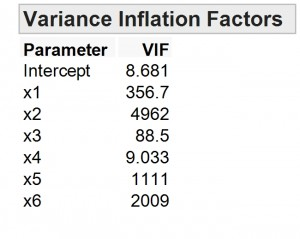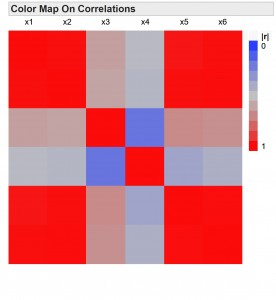Choose Language Hide Translation Bar
New in JMP 10 for experiment design: Evaluate Design

JMP 10 is coming in March. In my next few posts, I plan to share the main new capabilities in the area of experiment design. The most visible of these new features is the Evaluate Design item on the DOE menu.

What does the Evaluate Design feature do?

Evaluate Design allows you to see the design diagnostics for any data table as if it were a designed experiment.

Why would anyone want to do that?

I have heard two common reasons for wanting this feature. First, there are many designs in textbooks, and it is desirable to compare the capabilities of the textbook design to the algorithmic design that JMP produces. Second, it often happens that an analyst gets data from a colleague and wants to find out whether the data can adequately support various model possibilities.

The data in the table below was reported by Longley in the Journal of the American Statistical Association in 1967. The data is econometric data. The response, Y, is a measure of total employment. The columns X1 through X6 are the factors. You could probably guess, for instance, that X6 is actually calendar year.One thing about econometric data is that the variables are often correlated. Of course, this data is not the result of a designed experiment. Nevertheless, we can use design diagnostics to show why it is really hard to determine which of the six factors is actually driving the response.

You can find the Longley data in the file Longley.jmp in JMP’s Sample Data folder. The first thing we see after enter X1-X6 as factors and Y as the response in the launch dialog is a Fraction of the Design Space Plot. Points on the blue line in the plot show the fraction of the volume covered by the data that has a relative variance of prediction less than or equal to the plotted value. For instance, the vertical line falls on the X-axis at 0.5. The horizontal line intersects the vertical line at a point on the blue curve and hits the Y-axis at around 100. The interpretation of this is that half of the volume of space covered by the data has a relative variance of prediction less than 100. Conversely, half of the volume also has a relative variance of prediction greater than 100.So is that bad or good?

Actually, it is terrible! There are 16 rows in the table. If you could control X1 through X6 and perform an optimal design, the worst relative prediction variance would be 0.4375. So, in half of the region of the Longley data we are doing more than 200 times worse in prediction variance than the most poorly predicted combination of factors from a well-designed experiment.

The table below shows the VIF (variance inflation factors) for estimating coefficients for the main effects model. For an orthogonal design, the VIF for every coefficient is 1. We can see that because of the poor design of the data, the variance of the unknown parameters is as much as 2009 times worse than it would be for an orthogonal design.What is the cause of these poor diagnostics?

Opening the Color Map On Correlations outline node in the Evaluate Design report reveals the figure below. It is easy to see that X1, X2, X5 and X6 are nearly perfectly correlated. High correlations among factors results in high coefficient variance and high prediction variance.Economists have to deal with econometric data as it comes. Governments do not run designed experiments on national economies. Looking at these diagnostics we can appreciate why it is difficult to say why economic indicators move as they do.

One reason Longley wrote his paper was to emphasize the difficulties in interpreting multiple regression output with historical data like this. Happily, drawing conclusions from well-designed experiments is much simpler.

Article Labels

There are no labels assigned to this post.

Article Tags